## Solution to 1986 Problem 99

 The general formula for the binding energies of two oppositely charged objects orbiting each other with charges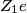$Z_1 e$ and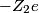$-Z_2 e$ and masses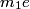$m_1 e$ and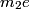$m_2 e$ are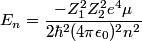\begin{align*}E_n = \frac{-Z_1^2 Z_2^2 e^4 \mu}{2 \hbar^2 (4 \pi \epsilon_0)^2 n^2}\end{align*} where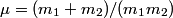$\mu = (m_1 + m_2)/(m_1 m_2)$ is the reduced mass of the pair. In this case,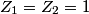$Z_1 = Z_2 = 1$ and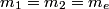$m_1 = m_2 = m_e$, so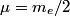$\mu = m_e/2$. So, the energies for postronium are equal to the energies of the hydrogen atom divided by two.